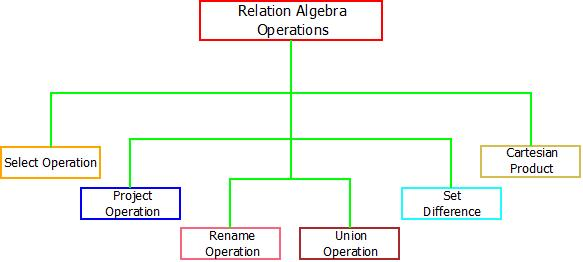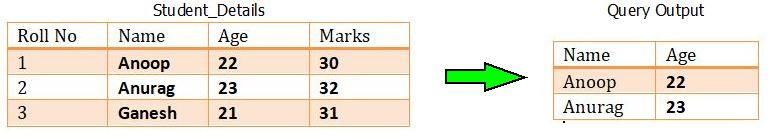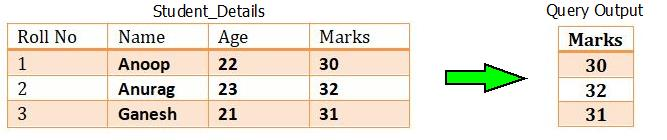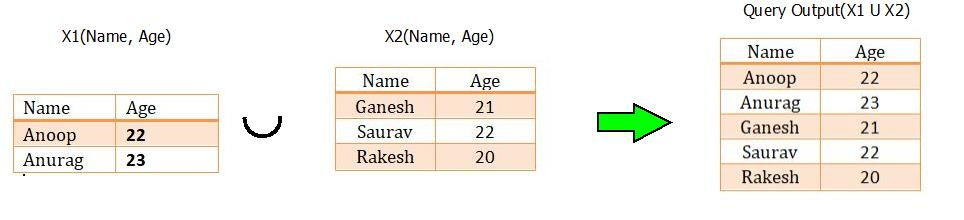# RELATIONAL ALGEBRA DBMS

Relational Algebra | DBMS Tutorial | Minigranth

# Relational Algebra : Introduction

• The relational algebra provides basic operations which can be performed over single or multiple relations in order to generate new relations(single or multiple). Relational algebra is a procedural query language which follows a particular syntax with the help of which, data can be accessed and retrieved very easily from single as well as multiple table/data sources.
• Certain operators are used to perform queries and retrieve desired results. These operators can perform certain operations on single attribute(called unary operator) or multiple attribute(called binary operator).
• There are six different types of operator that can be used to perform different operations. All of these are listed and explained below.Relational Algebra : Operations

### Relational Algebra : Operations

1. The Select Operation : This operation is used to fetch rows from given table or relation on the basis of given conditions, it is denoted by “Sigma(σ)”.

 Syntax : σ (Relation Name)

Here, “σ” is the select operation symbol. R is the relation from which the data needs to be fetched on the basis of conditions. Also, relational operators such as =, <, > etc. can also be used along. Let’s look at the example to get a clear picture of this.

For example : Consider the table of relation R(Roll No, Name, Age, Marks). If we want to select the name and age of student, then it can be done by:

Query Used : σ Name and Age>21 (Student_Details)Relational Algebra : Select Operation

2. The Project Operation : This operation is also used to fetch all the rows/tuples/data according to the requested attribute. It means, using project operation one can simply fetch all the tuples corresponding to a single attribute or multiple attributes. It does not supports any conditions as select operation and is denoted using “Pie(π)”.

 Syntax : π(Relation Name)

For example : Consider the table of relation R(Roll No, Name, Age, Marks). If we want to project the marks column, then it can be done by :

Query Used : πMarks(Student_Details)Relational Algebra : Project Operation

3. The Rename Operation : When operations like project and select are performed to fetch new results, these results requires renaming. They can be renamed using the rename operation which is denoted using Greek letter “Rho(ρ)”.

 Syntax : ρ(New Relation)

4. The Union Operation : In order to fetch data from two relations to generate new relation with combined capabilities, union operations can be used. The union operation fetches the data from both tables and projects it accordingly. It is denoted through “Union Symbol(U)”. Also, two things need to keep in mind while applying union operation are :

• Both the relations compulsory to have same number of attributes.
• Both the relations compulsory to have same domain for attributes.
 Syntax : X1 U X2 , where X1 & X2 are two different relations satisfying the above two conditions.

For example : Consider the two tables with relations X1(Name, Age) and X2(Name, Age). If we wish to apply the union operation, then it can be done by :Relational Algebra : Union Operation

5. The Set Difference Operations : In order to fetch the data which is not present in any one of the relation, set difference operation is used. The set difference operation is denoted by “Minus(-)”.

 Syntax :  X1 - X2  or X2 - X1 , where X1 & X2 are two different relations having some attributes.

Note : X1 - X2  ≠ X2 - X      {Not Commutative}

For example : Consider the two tables with relations X1(Name, Age) and X2(Name, Age). If we wish to apply the set difference operation, then it can be done by :Relational Algebra : Set Difference Operation

6. Cartesian Product : The Cartesian product operation will generate the possible combinations among the tuples from the relations resulting in table containing all the data. It combines the information of two or more relations in one single relation. Cartesian product is different from union operation and is denoted by “Cross(X)”.

 Syntax : A1 - A2 , where A1 & A2 are two different relations having some attributes.

For example : Consider the two tables with relations A1(Name, Roll No)and A2(Name, Roll No). If we wish to apply the Cartesian product operation, then it can be done by :Relational Algebra : Cartesian Product Operation